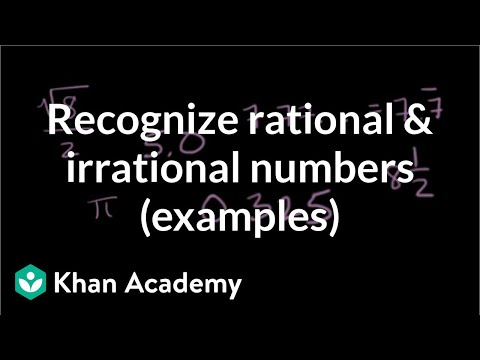Video

# Intro to square roots (Full video)

Description: Learn about the square root symbol (the principal root) and what it means to find a square root. Also learn how to solve simple square root equations. And now that we know a little bit about exponents, we'll see that the square root symbol or the root symbol or the radical is not so hard to understand. Well, that's the same thing as three times three and that's going to be equal to nine. So, we could write the square root of nine, and when you look at this way, you say, okay, what squared is equal to nine?

### Other videos you might be interested in### Classifying numbers (Full video)